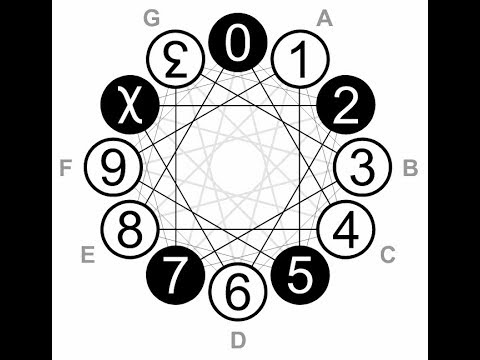I watched a video yesterday on base-6 aka seximal. It came up on HN.Cool stuff. The one sticking point I have is clocks: how are you going to use a base-12 clock in base-6? We put up with it now, with separators for the digits (12:59 vs 1L9 or whatever).

• 86,400 seconds in a day = S
• S / 6 = 14,400
• S / 62 = 2,400
• S / 63 = 400

And 400 seconds is about 7 minutes. You could keep going further and further into base-6 fractions, you’ve just got to shift the second to a power of 6. 63 = 216 is a lot closer than 64 = 1,296. So you just say a seximal second is 400/216 = ~1.85 seconds. And on we go.

• 86,400 * 216/400 = 46,656 seximal-seconds = 66
• 66 s-seconds = X
• X / 6 = 65 = 7,776 (?) in a day
• X / 62 = 64 = 1,296 s-minutes in a day
• X / 63 = 63 = 216 (?) in a day
• X / 64 = 62 = 36 s-hours in a day
• X / 65 = 61 = 6 (?) in a day

So it’s 12:04pm right now. In base-6, with weird 1.85-second “s-seconds” and so-forth (I’ll think of a cool name later, :P don’t worry), well let’s see: I’m 43,200 + 4x60 seconds into the day so far, which comes to 23,457.6 ssec. In base-6, that number is 300333.33… . The next “minute”, 12:05pm, would be 300430. Numbers instead of “o’clock” -type stuff.

I guess I should drop off the s-seconds part to be more equal comparisons. It’s “12:04pm” vs “3003” and “12:04:00pm” vs “300333”. Or “30:03:33”, whatever suits your fancy.

Whatever. It’s about as good as dozenal. The fractions definitely turn out better, as you can see in the video.